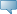# Lebanese American University WWW Information System

## Catalog Entries

Date
Dec 08, 2023Select the Course Number to get further detail on the course. Select the desired Schedule Type to find available classes for the course.

 MTH 207 - Discrete Structures I This course covers the foundations of discrete mathematics as they apply to computer science. The course is an introduction to propositional logic, logical connectives, truth tables, normal forms, validity, predicate logic, universal and existential quantification, and the limitations of predicate logic. Also, the following topics are covered: the number system, the Euclidean algorithm, proof techniques, mathematical induction, counting arguments, permutations and combinations, binomial coefficients, sets, functions, relations, matrices, and Boolean Algebra. Course Learning Outcomes: 1) Students shall be able to work with sets, relations, functions, and matrices. 2) Students shall be able to use different proof techniques including truth table, contradiction and induction. 3) Students shall be familiar with Boolean algebra. 4) Students shall be familiar with various counting principles. Prerequisite: MTH102 (can be taken concurrently) 0.000 TO 3.000 Credit hours 3.000 Lecture hours Levels: Undergraduate Schedule Types: Lab, Lecture, Tutorial Computer Science & Mathematics Department